Math Minion
A numerical problem solver for the

### NOTE!

I have ceased offering iOS and Mac versions of Math Minion in the Apple App store and am now only maintaining the web browser based version, which has its own domain name and site. This means Math Minion is now available on any platform that has a fairly recent web browser. It is now also completely open source, with the code available on GitHub.

This site is being maintained for archival purposes.

Math Minion started out as a powerful scientific calculator and modelling system with a deep understanding of conversion units, but over time it has evolved into much more. When string handling was introduced to its powerful array handling, it became an adept string processor. The addition of data tables allowed it to handle many small database and spreadsheet problems and the introduction of HTML interface objects now makes it something of an app minion. The journey continues.

It combines a mind map style representation of the relationships between expressions, with a formula system that operates transparently on scalars, arrays and matrices. A hierarchical model system helps encapsulate calculations in logical and easily duplicated units and a flexible unit conversion system tracks the fundamental property types through calculations. This allows it to know, for instance, that some fathoms divided by a few days is in fact a velocity that should not be added to a kilogram.

Some interesting features include:

• equations that work equally well with scalars, arrays and matrices
• a function library that in addition to the usual suspects includes:
• matrix multiplication, inversion, eigenvalues, eigenvectors, transpose and redimensioning
• comparison (eq, ne, lt, ge, if etc)
• string functions (split, join, regular expressions, etc.)
• interpolation, indexof, select
• complex number and statistical functions
• time, sorting, random, web reading and many more.
• an innovative unit conversion system with automatic dimensional analysis (Units slideshow)
• data tables for storing and manipulating tabular data whether numeric or string
• models and model arrays for information and calculation encapsulation
• a solver for one or more nonlinear equations
• an optimizer
• an ordinary differential equation solver
• an iterator tool.
• two and three dimension graphs.
• an HTML interface object for creating calculation user interfaces.
• share sessions between iOS and Mac devices using iCloud

Math Minion is a universal app that runs on the iPhone and iPod Touch as well as the iPad.

A completely compatible version for MacOS is also available for free in the Mac App Store.

All versions support iCloud document handling, so you can easily switch from device to device while working on the same sessions.

For more information, have a look at the manual from the application and a tutorial.

Free in the
iOS App Store
and Mac App Store

Screenshots (tap to expand)

Pendulum Model(On an iPad)

Based on a simple pendulum's mass (m), length (l), initial angle and velocity (y0), its motion is calculated. If the constant c is greater than zero, the motion will be damped.

3D Graph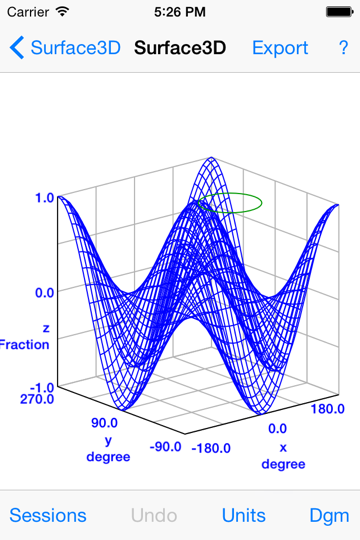(On an iPhone 4s)

A 3D surface graph and a 3D line graph (the circle).

Create HTML Interfaces(On an iPhone 6)

An HTML page tool is used to wrap a simple calculation with a user interface. It takes height and weight and uses them to calculate a body mass index. Note the use of mixed units in the inputs.

Optimizer(On a Mac)

A graph showing the results of using the optimizer to fit a group of randomized points with a parabola.

Data Tables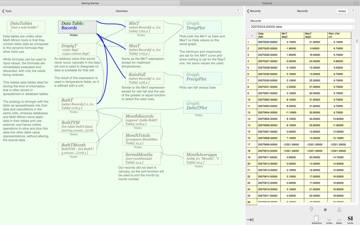(On a Mac)

Data, including strings, can be stored in data table tools. Other tools allow this to be selected and manipulated in many ways.

Not Just for Science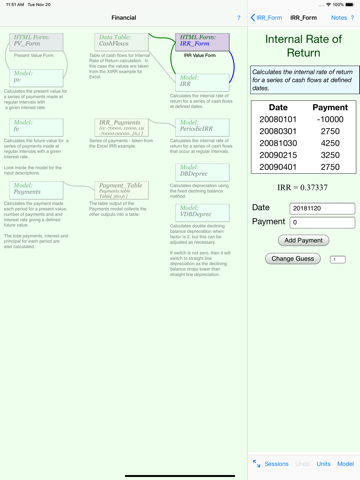(On an iPad)

Math Minion also has a range of financial functions as well as advanced string handling including regular expressions. In this shot from a session on the Examples page, an HTML page tool is used to display an internal rate of return calculation.

Formula Editing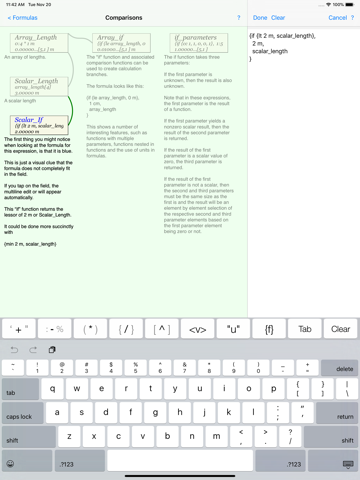(On an iPad)

There is an expanded keyboard when editing formulas on iOS and it includes buttons for browsing conversion units, functions and objects and their contents. A tap will insert whatever you choose into the formula.

Here the formula field has been expanded to allow a multiline formula to be entered, which uses the if and gt conditional functions to limit the size of a value to 2 m. If used with an array, each element would be limited.

Steam Turbine Calculation(On an iPad)

Math Minion can be used for quite complex calculations such as this steam turbine calculation that uses models that implement the Steam 97 from IAPWS. The models are available on the Examples page and can be cut and pasted into new sessions as they have been here.

(Yes, Math Minion can also perform complex calculations as in the square root of minus one kind).

McCabe Thiele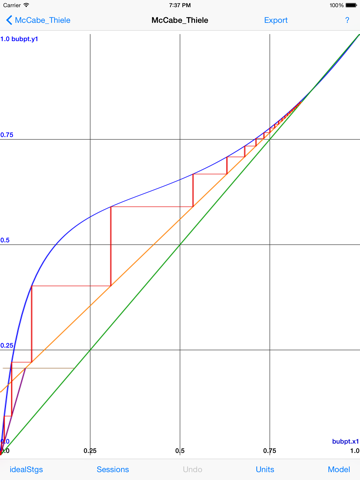(On an iPad)

A McCabe Thiele distillation column diagram is constructed on an ethanol water equilibrium diagram calculated using Margules.

Please see the Examples page as well.